lvy>試卷(2022/08/12)

# 106 年 - 106 國立中山大學_碩士班招生考試_電波領域：電子學#110216

【非選題】
1.

1.(30%) Figure 1 shows a Colpitts oscillator. Assume β=100,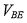= 0.7 V, and VT = 25 mV for BJT

Q.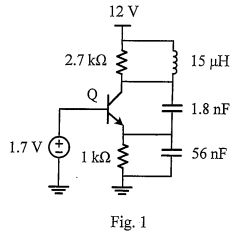【題組】

(a)(10%) Estimate the quality factor of the equivalent smnall-signal LCR circuit seen at the collector

of Q.

【非選題】
2.【題組】

(b) (10%) If the sustained oscillation occurs, what will be the oscillation frequency (in Hz)?

【非選題】
3.【題組】

(c)(10%) Calculate the small-signal transconductance gm of the transistor Q, and determine if the

feedback gain is large enough to cause oscillation. Justify your answer.

【非選題】
4.

2. (20%) Suppose we want to convert v(t)=cos(10t) to v(t) = Axsin(10t) by a phase shift circuit in Fig. 2. Determine the value of R, and what will be the output amplitude A in this case?【非選題】
5.

3. (20%) A series RC circuit is utilized to equalize the impedance of an inductive load in Fig. 3. Determine the value of C in Fig. 3 so that the overall impedance Z becomes a pure resistance of 50 ohm in the entire frequency band.【非選題】
6.

4. (30%) Figure 4 shows a MOSFET transistor M that makes a constant current source for a 20-kΩ load. The body terminal and source terminal of MOSFET M are tied together. Assume that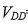= 15 V, and the transistor M has the device parameters: WIL = 80,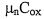= 50 μA/V2,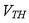=0.7 V,λ = 0.1. Suppose we want to have ID = 0.5 mA.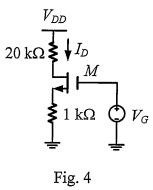【題組】(a) (10%) Determine VG.

【非選題】
7.【題組】

(b) (10%) Determine the minimum allowedthat still assures that M works properly as a constant current source.

【非選題】
8.【題組】

(c) (10%) Suppose the 15V supply voltage has 1% variations on it (i.e.,△=± 0.15V). Estimate the percentage change in current Io. Notefor M in saturation.

### 懸賞詳解

#### 國一公民下第三次

36. 颱風天強風豪雨不斷，挾帶土石流肆虐，造成道路坍方，民眾房屋淹水。政府最可能對受災 戶採取下列何種措施？ (A)提供就業諮詢 (B)推動無障礙�...